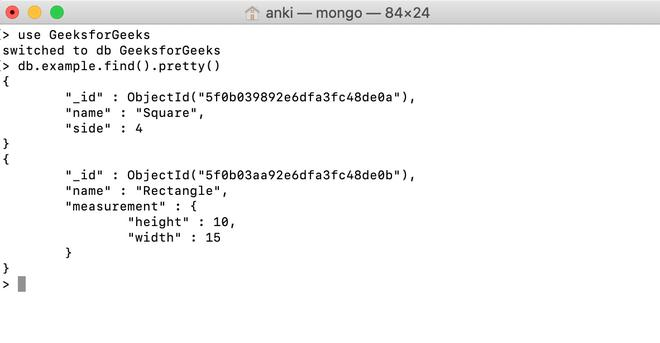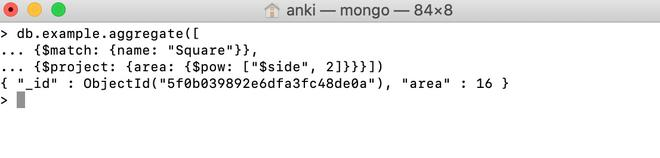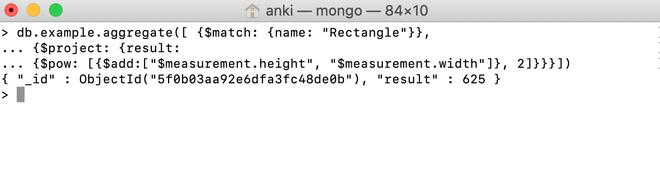GFG App
Open AppBrowser
Continue

MongoDB provides different types of arithmetic expression operators that are used in the aggregation pipeline stages \$pow operator is one of them. This operator is used to find a number to the specified exponent and returns the result.

Syntax:

```{ \$pow: [ <number>, <exponent> ] }

```

Here, the number and exponent are the valid expressions until it resolves to a number.

• If the entered value resolves to null, then this operator will return null.
• If the entered value resolves to NaN, then this operator will return NaN.
• If the entered value refers to a missing field, then this operator will return null.

The result will be in the same type as the input, but it can be changed when it cannot be represented accurately in that type like in the following cases:

• The 32-bit integer will be converted to a 64-bit integer if the result is represented as a 64-bit integer.
• The 32-bit integer will be converted to a double if the result is not represented as a 64-bit integer.
• The 64-bit integer will be converted to double if the result is not represented as a 64-bit integer.

Examples:

In the following examples, we are working with:

Database: GeeksforGeeks

Collection: example

Document: two documents that contain the details of the shapes in the form of field-value pairs.Using \$pow Operator:

In this example, we are going to find the area of square (i.e., (side)2 ) using a \$pow operator. Here, the \$pow operator contains two arguments, first one is the value of side field(i.e., 2) and the second one is the exponent(i.e., 2).

```db.example.aggregate([
... {\$match: {name: "Square"}},
... {\$project: {area: {\$pow: ["\$side", 2]}}}])

```Using \$pow Operator in the Embedded Document:

In this example, we are going to find the value of (measurement.height +measurement.width)2  using a \$pow operator. Here, \$pow operator contains two arguments, first one is the sum of the values of measurement.height and measurement.width fields and the second one is the exponent(i.e., 2).

```db.example.aggregate([ {\$match: {name: "Rectangle"}},
... {\$project: {result: